# Analytics Calculations: Multi-Tiered Sorting 2

Moderator, Lightspeed Staff Posts: 66 moderator
What if we want to sort by two sets of data, but unlike our first Multi-Tiered sorting, they are not both numerical? Dates can be converted to numbers easily, but what if we're looking at non-numeric dimensions?

Let's say that we want to rank categories of sales at each of our Multi-Store locations...

# Option 1

## Concat Store Name and Sales Totals

Remember from our cell-based functions that we can use the "Concat" function to gather content from two or more cells and then string them together...

Now if I just concat the name of the shop and the sale line total...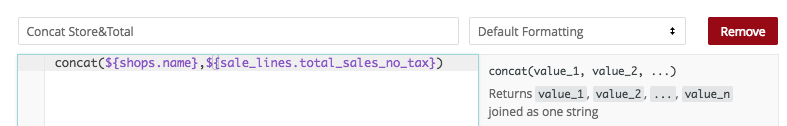I'll get a new value for sorting, but...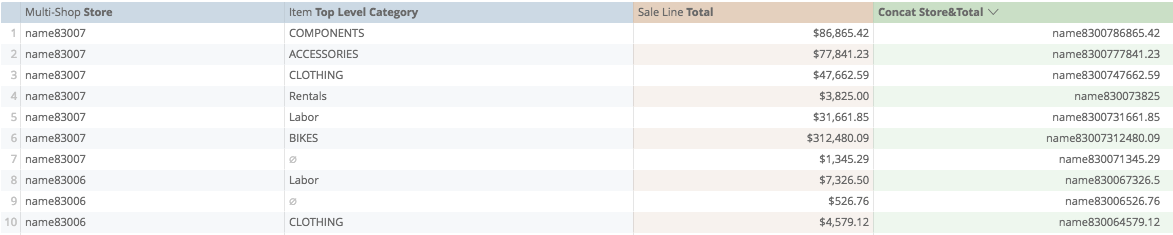...it doesn't sort the totals as expected.

Do you see why?

Right! Now that the sales totals numbers have been turned to text, they are sorted by their first digit, not by their total. As we see above, 38 is greater than 31, so \$3,825 is considered higher than \$31,662.

What we need to do is create a protocol for keeping the value of the totals. So that in the example above, 3,800 and 31,000 become 03825 and 31662.

This is easier than it sounds.

What we could do is add the same really high number to each of the totals, that way, their values are still in order, but the initial digit is less important.

Our top category here is six digits long, so if we were to add an eight digit number to everything, the numeric and text sorting would work as expected.

So let's start by adding 10,000,000 to each Sale Line total...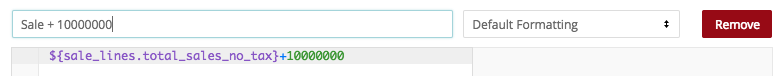Now, when I save...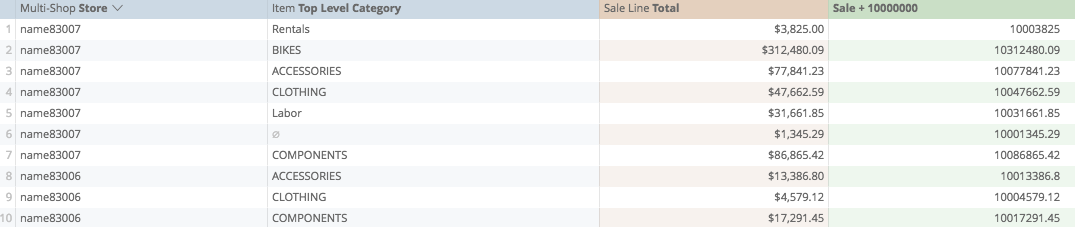3,852 becomes 10,003,825, 312,480 becomes 10,312,480, and so on, so now can start our concat function...

We'll start a new calculation, and again from our best practices, start by creating the function just with brackets...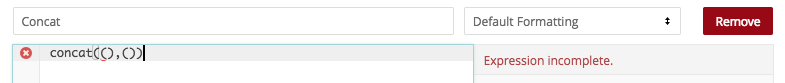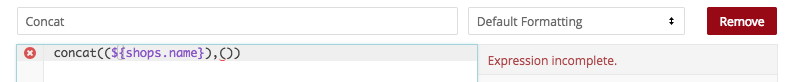...then we'll copy the calculation from our first field...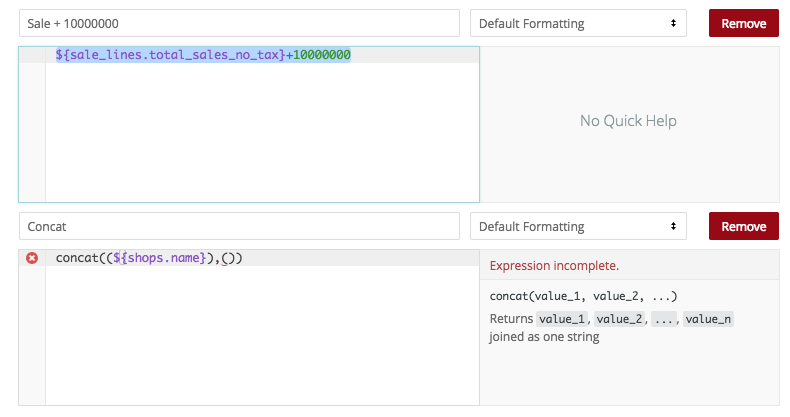...and paste it into the second set of brackets...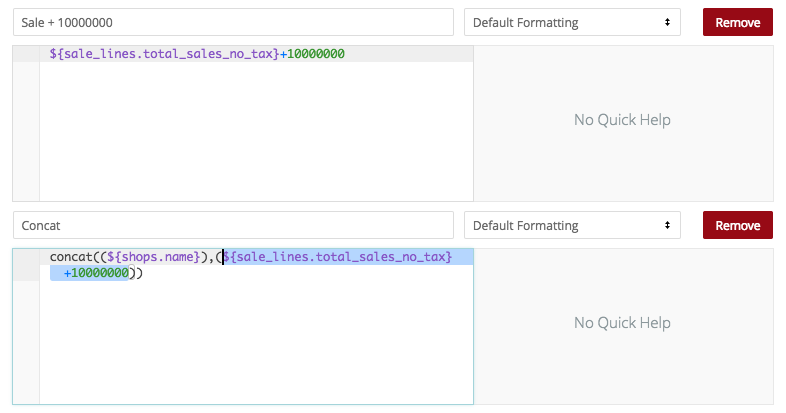So, when I save...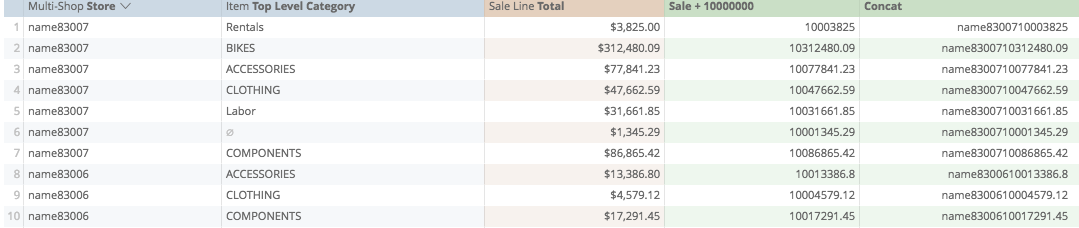Now our number sequence is preserved, so that when we sort...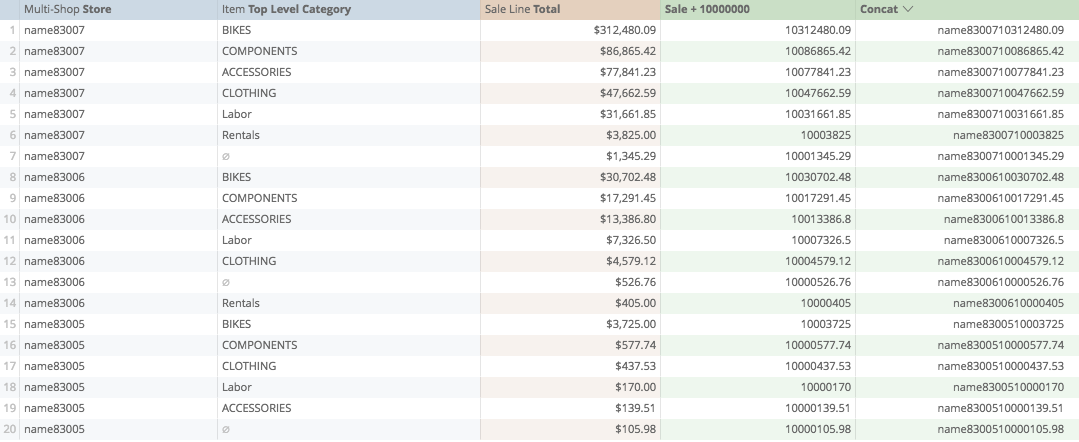our store grouping and sorting by dollar amount is preserved!

Now, because our first calculation is implicit in the second, we can remove the first, and we can also hide our sorting calculation from visualization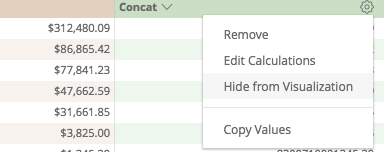So that our final report only shows us the dimensions and measures that we want!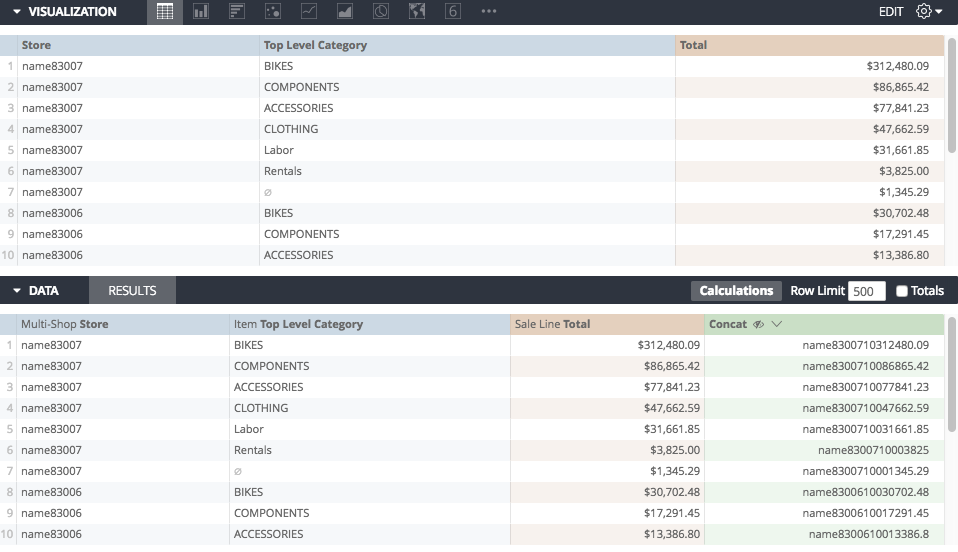In this instance, our final calculation is:

concat((\${shops.name}),(10000000+\${sale_lines.total_sales_no_tax}))

# Option 2

## Convert Stores to Numbers

We could also use IF functions to change the names of our stores into numbers for the purpose of sorting.

The advantage to using this method is that you can custom-sort the stores instead of using Alphabetical sorting.

A drawback to using this method is that you will need to update the calculation as new stores are added to your list.

Similarly to our date sorting from the first method, we want to use really high numbers to as the basis of the store, and we want the highest stores in our ranking to have the highest numbers.

This is how it works:

Say I have three stores, and I want to focus on Store 6 first, in my IF statement, I will identify Store 6 as 30,000,000...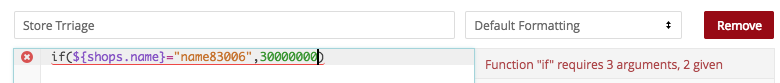Then, I can look at my next store, perhaps store 5, and I will assign to it, 20,000,000...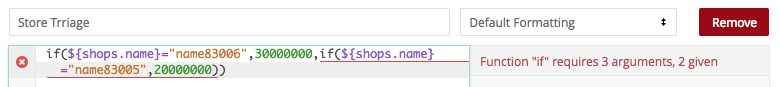Then I could either define Store 7 as being 10,000,000, or I could define everything else as 10,000,000 (in this instance, there is only one other thing to be "everything else"; Store 7.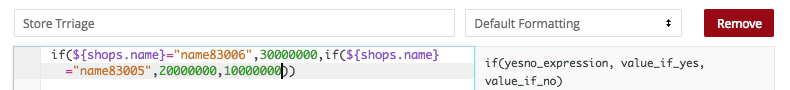So now when I save, I have a calculation showing me just the numbers...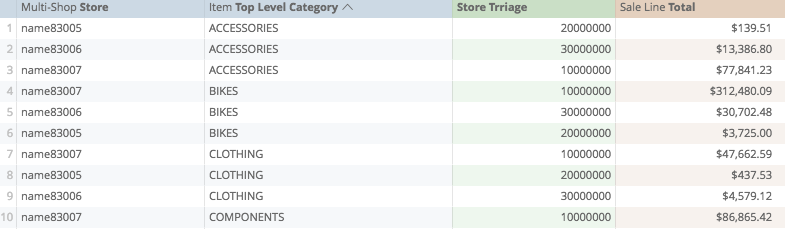Now, because the stores are numbers, we can add the sales total to this number for our final sorting.

Again, we start by creating the base of the calculation with brackets...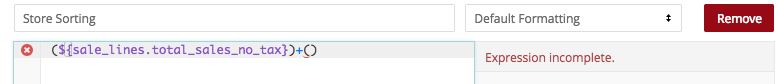...select the calculation from above...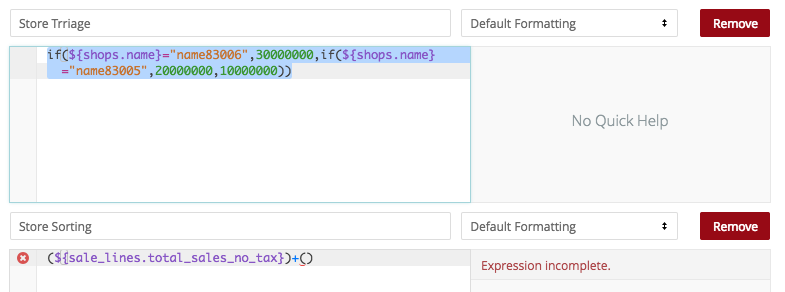and paste it into the calculation below...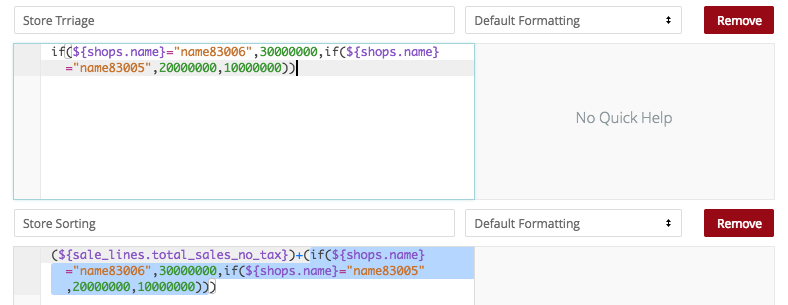This gives us a final calculation creating the store and sales total as one number...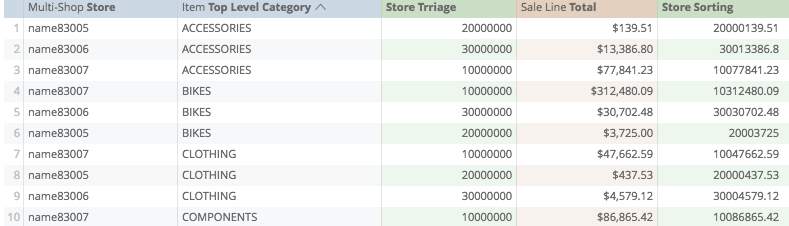So that when we sort...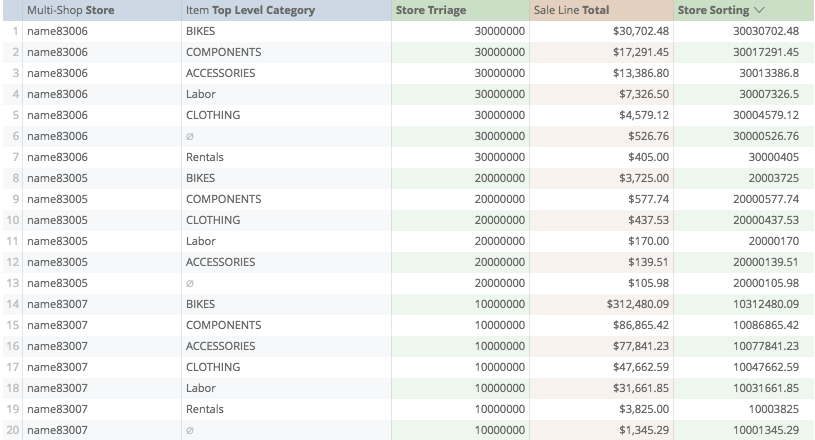Our stores and sales totals are kept in the order we want!

Similarly, we can remove the first calculation, and hide the last calculation...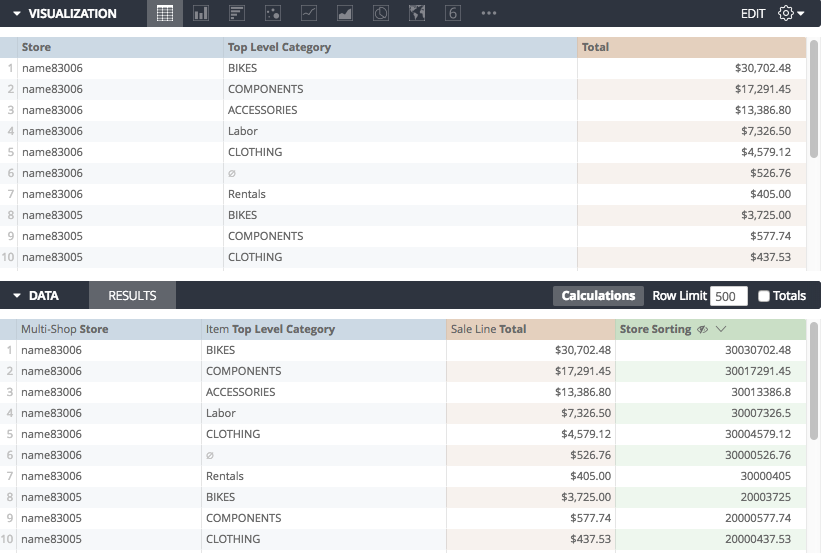So that our final report keeps the sorting calculation hidden!

*Note if we really want to make these reports dynamic, we could use the "length" function to identify how many characters are in a Sales Total field, use the "large" function to identify the largest number from these, add two to this number, and then create our "really large" number by multiplying 10 to the power of that number. This way, as sales totals grow in time, the triage number follows it, always to be untouched by the sales totals

Calculations Home
Joseph McKeown
Lightspeed Analytics and reporting consultant
Lightspeed HQ